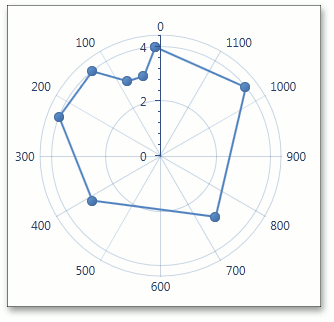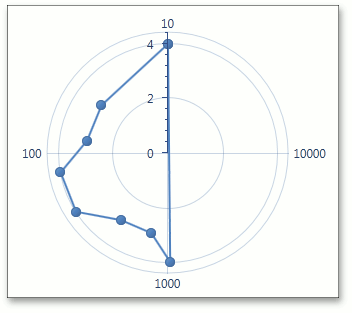21.2
21.1
20.2
20.1
19.2
19.1
18.2
18.1
17.2

Gets or sets whether the radar X-axis should display its numerical values using a logarithmic scale.

Namespace: DevExpress.Xpf.Charts

Assembly: DevExpress.Xpf.Charts.v21.2.dll

## Declaration

``public bool Logarithmic { get; set; }``

## Property Value

Type Description
Boolean

true to display the logarithmic scale; otherwise, false.

## Remarks

If a chart’s series has its Series.ArgumentScaleType property set to ScaleType.Numerical, you can use the Logarithmic property to specify whether a logarithmic scale should be used to display axis values. When the Logarithmic property is set to true, you can also define a logarithmic base value via the RadarAxisX2D.LogarithmicBase property.

Usually, the Logarithmic property is used when the data range of a chart’s series has significant disparity. By enabling this property, the chart’s numerical axes display all values using logarithmic equivalents. In short, if the logarithmic base is 10, only 5 uniform axis steps will exist between 10 and 1,000,000.

The following table illustrates the Logarithmic property in action.

Logarithmic = false

Logarithmic = true

LogarithmicBase = 10